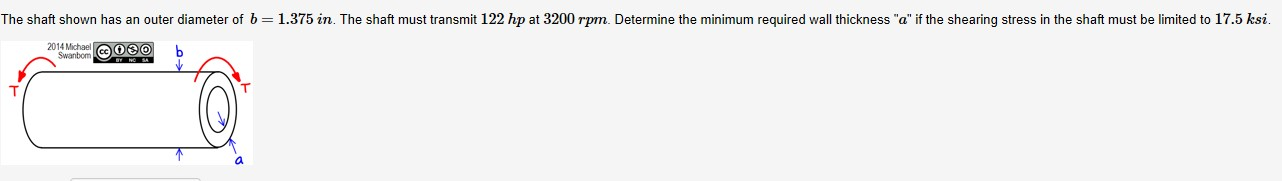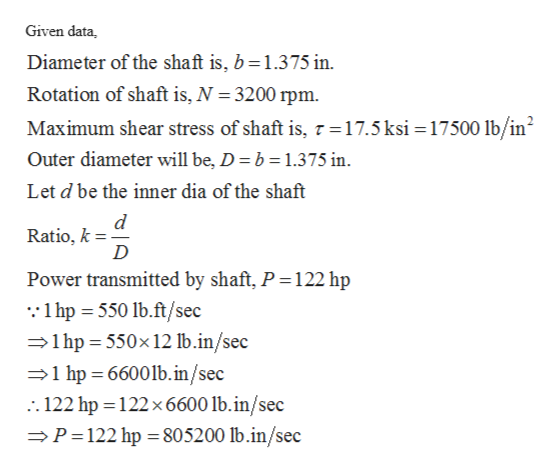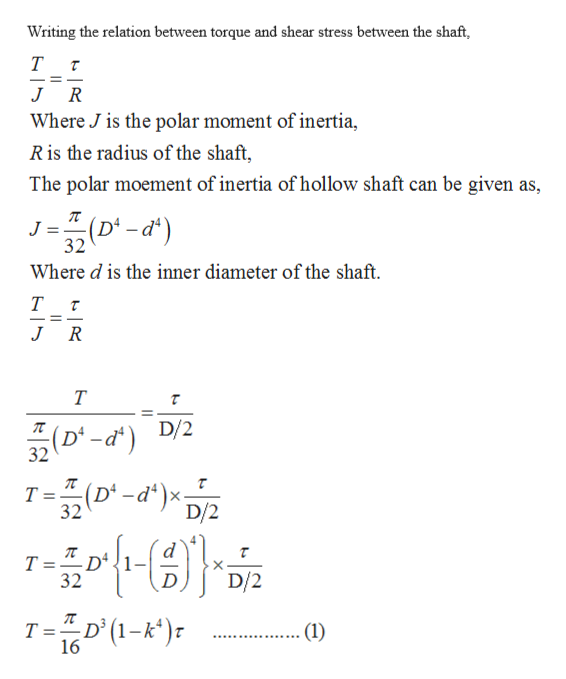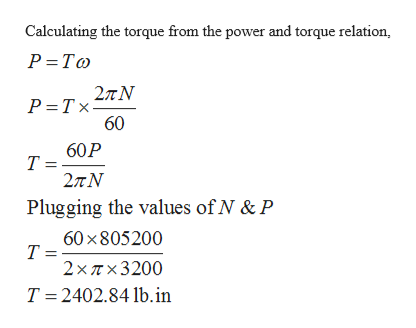# The shaft shown has an outer diameter of 6 = 1.375 in. The shaft must transmit 122 hp at 3200 rpm. Determine the minimum required wall thickness "a" if the shearing stress in the shaft must be limited to 17.5 ksi.2014 MichaelSwanbom eOe0

Question
4 views

Problem 5help_outlineImage TranscriptioncloseThe shaft shown has an outer diameter of 6 = 1.375 in. The shaft must transmit 122 hp at 3200 rpm. Determine the minimum required wall thickness "a" if the shearing stress in the shaft must be limited to 17.5 ksi. 2014 Michael Swanbom eOe0 fullscreen
check_circle

Step 1help_outlineImage TranscriptioncloseGiven data, Diameter of the shaft is, b=1.375 in. Rotation of shaft is, N = 3200 rpm. Maximum shear stress of shaft is, 7 =17.5 ksi =17500 lb/in? Outer diameter will be, D = b = 1.375 in. Let d be the inner dia of the shaft Ratio, k = D Power transmitted by shaft, P =122 hp :1 hp = 550 lb.ft/sec =1hp = 550×12 lb.in/sec =1 hp = 6600lb.in/sec .. 122 hp = 122x6600 lb.in/sec =P=122 hp =805200 lb.in/sec fullscreen
Step 2help_outlineImage TranscriptioncloseWriting the relation between torque and shear stress between the shaft, т J R Where J is the polar moment of inertia, R is the radius of the shaft, The polar moement of inertia of hollow shaft can be given as, J=(D' -d*) %3D 32 Where d is the inner diameter of the shaft. R 32(D-a*) D/2 т (D' -d*)×; 32 %3! D/2 - Dª T =. D/2 32 T =D°(1-k*)r (1) 16 fullscreen
Step 3help_outlineImage TranscriptioncloseCalculating the torque from the power and torque relation, P =To 2лN P =Tx= 60 60P 2πΝ Plugging the values of N & P 60 x805200 2x Tx3200 T = 2402.84 lb.in fullscreen

### Want to see the full answer?

See Solution

#### Want to see this answer and more?

Solutions are written by subject experts who are available 24/7. Questions are typically answered within 1 hour.*

See Solution
*Response times may vary by subject and question.
Tagged in